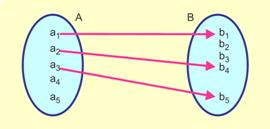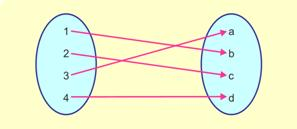# Short Notes: Relations and Functions# Relations

#### Relation: Meaning

Let A and B be two sets, then a relation R from A to B is a subset of A ×B.

Thus, R is a relation from A to B . ⇔  R ⊆ A × B

Recall that A × B is a set of all ordered pairs whose first member is from the set and second member is from i.e.,

A × B = {(x,y) : x  A and y ∈ B }

If R is a relation from a non-empty set A to a non-empty set and if (a, b) ∈ R, then we write aRb which is read as is related to by the relation R. If (a, b) ∉ R then we say that a is not related to b by the relation R. In other words, a relation is a set of inputs and outputs, often written as ordered pairs (input, output). We can also represent a relation as a mapping or a graph. For example, a relation can be represented as:Mapping diagram of Relation

Domain and Range of relation: Let R be a relation from a set A to set B. Then the set is called the domain of R. The set of all second elements of the ordered pairs in R is called the range of R. The set is called the co-domain of the relation R.

# Types of Relations

(i) Universal Relation : If A be any set, then  R = A × A ⊆ A × A and so it is a relation on A. This relation is called the universal relation on A.

(ii) Empty (or Void) Relation : If A be any set, then Φ ⊆ A × A  and so it is a relation on A. This relation is called Empty (or void) relation.

(iii) Reflexive Relation : A relation R is said to be reflexive, if every element of A is related to itself.

Thus, R is reflexive ⇔ (a,a) ∈ R, for all  a ∈ A .

(iv) Symmetric Relation : A relation R on a set A is said to be a symmetric if (a,b) ∈ R ⇔ (b,a) ∈ R for all a,b ∈ A

(v) Transitive Relation : A relation on any set A is said to be transitive if (a,b) ∈ R, (b,c)∈ R ⇒(a,c)∈ R,for all  a,b,c ∈ A.

i.e.,     aRb and bRc ⇒ aRC   for all a,b,c ∈ aRC

Equivalence Relation : A relation R on a set A is said to be an equivalence relation on A if

(a) it is reflexive

(b) it is symmetric

(c) it is transitive

# Functions

Function : Meaning

Let A and B be two non-empty sets, then a rule f which associates each element of A with a unique element of B is called a function or mapping from A to B.

If f is a mapping from to B, we write  f : A → B (read as f is a mapping from to B).

If f associates x ∈ A to y ∈ B then we say that y is the image of the element under the map (or function) f and we write (x). And, the element x is called the pre-image of y.

### Types of Functions

(1) One-One Function (Injective) : A function f : A → B is said to be a one-one function or an injective if different elements of A have different images in B.

Thus, f : A → B is one-one.

⇔ a≠b  ⇒ f(a) ≠ f(b) for all a,b ∈ A or f(a) = f(b)⇔ a=b for all  a,b ∈ A(2) Onto-Function (Surjective) : A function f : A → B is said to be an onto function or surjective if and only if each element of B is the image of some element of A i.e. for every element y ∈ B there exists some x ∈ A such that (x). Thus f is onto if range of
= co-domain of f.(3) One-one and onto Function (Bijective Function) : A function is a bijective if it is one-one as well as onto# Composition of Functions

Suppose A, B and C be three non-empty sets and let  f : A → B,     g : B → C be two functions. since f is a function from A to B, therefore for each  x ∈ A there exists a unique element

f(x) ∈ B  Again, since g is a function from B to C, therefore corresponding to f(x) ∈ B there exists a unique element  g(f(x)) ∈ C Thus, for each  fx ∈ A there exists a unique element g(f(x)) ∈ C .

From above discussion it follows that when f and g are considered together they define a new function from A to C. This function is called the composition of f and g and is denoted by gof.

i.e. gof(x) = g((x)), for all x ∈ A# Inverse of a function

If and g be two functions satisfying
(g(x)) = x, for every x in the domain of g, and g(f(x)) = x for every in the domain of f.

We say that f is the inverse of g and also, g is the inverse of f. We write  f = g−1or g = f−1

Tips: To find the inverse of f, write down the equation yf(x) and then solve x as a function of y.

Thus the resulting equation is x = f−1(y) .

Note : (i) If f is one-one function then f has inverse defined on its range

(ii) If f and g are one-one function then  (fog)−1 = g−1of−1

(iii) If f is bijective then f−1 is bijective and (f−1)−1  = f.

## Binary Operation and Properties of Binary Operations

Binary Operation : A binary operation ∗ on a set is a function   ∗: A × A  . We denote ∗ (a, b) by ∗ b.

It follows from the definition of a function that a binary operation on a set associates each ordered pair (a, b) ,

(a,b) ∈ A × A to a unique element (a, b) in A.

Properties of Binary Operations

(i) Commutativity: A binary operation ∗ on a set of is said to be commutative binary operation, if

a ∗ b =b ∗ a   for all a,b ∈ A

The binary operations addition (+) and multiplication (×) are commutative binary operation on z.

(ii) Associativity: A binary operation ∗: A × A → Ais said to be associative if

(a∗b)∗c =a∗(b∗c) ,  ∀ a,b,c ∈ A

The binary operation of addition (+) and multiplication (×) are associative binary operation on z. However, the binary operation of subtraction is not associative binary operation.

(iii) Identity Element: Let ′∗′ be a binary operation on a set and if there exists an element e ∈ A, such that a∗e = a = e∗a,  for all a ∈ A, then is called an identity element for the binary operation ′∗′  on set and it is unique.

(iv) Inverse of an element: Let ′∗′  be a binary operation on a set A, and let be the identity element in for the binary operation ∗ on S.Then, an element  a ∈ A is called an invertible element if there exists an element b ∈ A such that a×b = e = b∗a.

The element is called the inverse of an element a, and it is unique.

Note: The inverse of a element is generally denoted by a-1